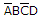Determine the values of A, B, C, and D that make the sum termequal to zero.

Which of the following expressions is in the sum-of-products (SOP) form?

The systematic reduction of logic circuits is accomplished by:

An AND gate with schematic "bubbles" on its inputs performs the same function as a(n)________ gate.

How many gates would be required to implement the following Boolean expression before simplification? XY + X(X + Z) + Y(X + Z)

Determine the values of A, B, C, and D that make the product termequal to 1.

What is the primary motivation for using Boolean algebra to simplify logic expressions?

How many gates would be required to implement the following Boolean expression after simplification? XY + X(X + Z) + Y(X + Z)

Which Boolean algebra property allows us to group operands in expression in any order without affecting the results of the operation [for example, A + B = B + A]?

When grouping cells within a K-map, the cells must be combined in groups of ________.